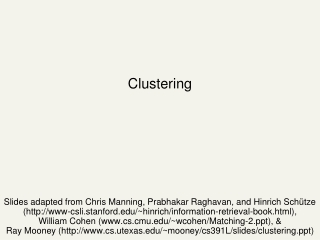DownloadDownload PresentationClustering

# Clustering

Download Presentation## Clustering

- - - - - - - - - - - - - - - - - - - - - - - - - - - E N D - - - - - - - - - - - - - - - - - - - - - - - - - - -
##### Presentation Transcript

1. Clustering Slides adapted from Chris Manning, Prabhakar Raghavan, and Hinrich Schütze (http://www-csli.stanford.edu/~hinrich/information-retrieval-book.html),William Cohen (www.cs.cmu.edu/~wcohen/Matching-2.ppt), & Ray Mooney (http://www.cs.utexas.edu/~mooney/cs391L/slides/clustering.ppt)

2. What is clustering? • Clustering: the process of grouping a set of objects into classes of similar objects • Most common form of unsupervised learning • Unsupervised learning = learning from raw data, as opposed to supervised data where a classification of examples is given

3. Clustering

4. Clustering

5. Clustering: Navigation of search results • For grouping search results thematically • clusty.com / Vivisimo

6. Clustering: Corpus browsing www.yahoo.com/Science … (30) agriculture biology physics CS space ... ... ... ... ... dairy botany cell AI courses crops craft magnetism HCI missions agronomy evolution forestry relativity

7. Clustering considerations • What does it mean for objects to be similar? • What algorithm and approach do we take? • Top-down: k-means • Bottom-up: hierarchical agglomerative clustering • How many clusters? • Can we label or name the clusters? • How do we make it efficient and scalable?

8. What makes docs “related”? • Ideal: semantic similarity. • Practical: statistical similarity • Treat documents as vectors • For many algorithms, easier to think in terms of a distance (rather than similarity) between docs. • We focus on Euclidean distance

9. What are we optimizing? • Given: Final number of clusters • Optimize: • “Tightness” of clusters • {average/min/max/} distance of points to each other in the same cluster? • {average/min/max} distance of points to each clusters center? • Usually clustering finds heuristic approximations

10. K-Means • Assumes real-valued vectors. • Clusters based on centroids (aka the center of gravity or mean) of points in a cluster, c: • Reassignment of instances to clusters is based on distance to the current cluster centroids.

11. K-Means Algorithm Select K random seeds. Until clustering converges or other stopping criterion: For each xi: Assign xi to the cluster cjsuch that dist(xi, sj) is minimal. (Update the seeds to the centroid of each cluster) For each cluster cj sj = (cj) How?

12. Pick seeds Reassign clusters Compute centroids Reassign clusters x x Compute centroids x x x x K Means Example(K=2) Reassign clusters Converged!

13. Seed Choice • Results can vary based on random seed selection. • Some seeds can result in poor convergence rate, or convergence to sub-optimal clusterings. • Select good seeds using a heuristic (e.g., doc least similar to any existing mean) • Try out multiple starting points • Initialize with the results of another method. Example showing sensitivity to seeds In the above, if you start with B and E as centroids you converge to {A,B,C} and {D,E,F} If you start with D and F you converge to {A,B,D,E} {C,F}

14. How Many Clusters? • Number of clusters K is given • Partition n docs into predetermined number of clusters • Finding the “right” number of clusters is part of the problem • Given data, partition into an “appropriate” number of subsets. • E.g., for query results - ideal value of K not known up front - though UI may impose limits. • Can usually take an algorithm for one flavor and convert to the other.

15. animal vertebrate invertebrate fish reptile amphib. mammal worm insect crustacean Hierarchical Clustering • Build a tree-based hierarchical taxonomy (dendrogram) from a set of documents. How could you do this with k-means?

16. Hierarchical Clustering algorithms • Agglomerative (bottom-up): • Start with each document being a single cluster. • Eventually all documents belong to the same cluster. • Divisive (top-down): • Start with all documents belong to the same cluster. • Eventually each node forms a cluster on its own. • Could be a recursive application of k-means like algorithms • Does not require the number of clusters k in advance • Needs a termination/readout condition

17. Hierarchical Agglomerative Clustering (HAC) • Assumes a similarity function for determining the similarity of two instances. • Starts with all instances in a separate cluster and then repeatedly joins the two clusters that are most similar until there is only one cluster. • The history of merging forms a binary tree or hierarchy.

18. Dendogram: Hierarchical Clustering • Clustering obtained by cutting the dendrogram at a desired level: each connected component forms a cluster.

19. Closest pair of clusters Many variants to defining closest pair of clusters • Single-link • Distance of the “closest” points (single-link) • Complete-link • Distance of the “furthest” points • Centroid • Distance of the centroids (centers of gravity) • (Average-link) • Average distance between pairs of elements

20. Single Link Agglomerative Clustering • Use maximum similarity of pairs: • Can result in “straggly” (long and thin) clusters due to chaining effect. • After merging ci and cj, the similarity of the resulting cluster to another cluster, ck, is:

22. Complete Link Agglomerative Clustering • Use minimum similarity of pairs: • Makes “tighter,” spherical clusters that are typically preferable. • After merging ci and cj, the similarity of the resulting cluster to another cluster, ck, is: Ci Cj Ck